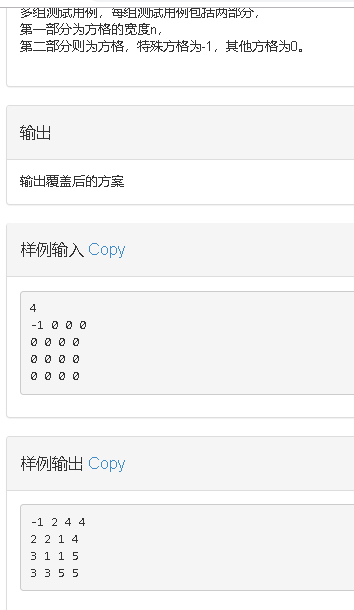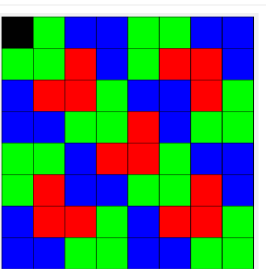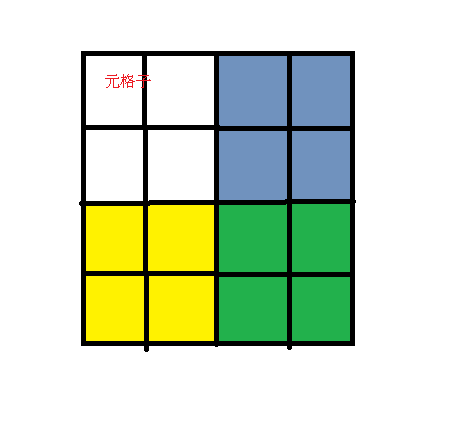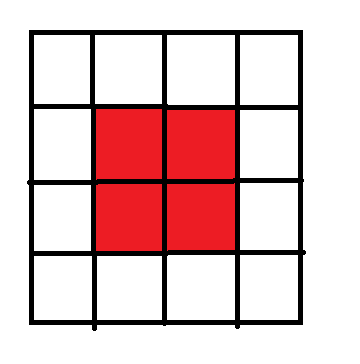# 棋盘覆盖之分治加递归

## 问题if条件里判断是否在左/右-上/下角正方形中的方法 ： 每次按照将小块的正方形的最左上角方格作为元格子，而if里面的chek特殊方格位置不变，改变元格子的位置到小正方形的元格子进行递归

 if(x1>=x+s&&y1<y+s)//左下角
{
chek(x+s,y,x1,y1,s);

}
else{
a[x+s][y+s-1]=t;
chek(x+s,y,x+s,y+s-1,s);
}


a数组 是将左下角的红色格子进行覆盖，所以是a[x+s] [y+s-1]else里面的chek 参数是将黄色正方形的第一个格子作为元格子，将上一行代码覆盖的格子作为特殊格子进行填写，而s照常

## 完整代码

#include<bits/stdc++.h>

using namespace std;
const int N=1005;
int a[N][N];
int titl=1;

void chek(int x,int y,int x1,int y1,int s2)
{
if(s2==1)
return;
int s=s2/2;
int t=titl++;
if(x1<x+s&&y1<y+s)//左上角
chek(x,y,x1,y1,s);//如果在此正方形内则继续递归
else{//如果不在此正方形内则覆盖构造一个特殊方格，构造左上角的对角为特殊方格
a[x+s-1][y+s-1]=t;//覆盖构造特殊格子
chek(x,y,x+s-1,y+s-1,s);
}
if(x1>=x+s&&y1<y+s)//左下角
{
chek(x+s,y,x1,y1,s);

}
else{
a[x+s][y+s-1]=t;
chek(x+s,y,x+s,y+s-1,s);
}
if(x1<x+s&&y1>=y+s){//右上角
chek(x,y+s,x1,y1,s);
}else{
a[x+s-1][y+s]=t;
chek(x,y+s,x+s-1,y+s,s);
}

if(x1>=x+s&&y1>=y+s)//右下角
chek(x+s,y+s,x1,y1,s);
else{
a[x+s][y+s]=t;
chek(x+s,y+s,x+s,y+s,s);
}
}
int main(){
int k;
while(cin>>k)
{
int dx,dy;
titl=1;
for(int i=0;i<k;i++){
for(int j=0;j<k;j++){
scanf("%d",&a[i][j]);
if(a[i][j]==-1)
{
dx=i;
dy=j;
}
}
}
chek(0,0,dx,dy,k);
for(int i=0;i<k;i++){
for(int j=0;j<k;j++){
printf("%d ",a[i][j]);

}
printf("\n");
}

}
return 0;
}


©️2019 CSDN 皮肤主题: 游动-白 设计师: 上身试试